Next: Conclusion Up: number9 Previous: The RLS filter

Application example - echo cancellation

As example of how to apply an adaptive filter to a real-world problem, lets consider the problem of echo cancellation. In this problem there is a signal that we want to recover,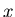, that we measure but it is contaminated with multiple copies of itself. These extra copies overlap with unknown delay times and amplitudes. Mathematically the measurements consist of the series,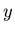, which is the convolution of the clean signaland the unknown reflection coefficient series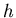,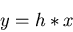(9)

The goal of the filter is to estimate the unknown reflection coefficients.

This type of problem is sometimes solved by direct mathematical inversion of the known quantitiesand. In the engineering literature this is sometimes called the system identification problem, in geophysics its known as deconvolution. An adaptive filter can be used to solve this problem in real-time, but it takes some amount of time for it to converge (particularly if the signal power is low). The adaptive filter approach will also work whenis slowly varying with time; this is something that standard deconvolution does not work well with.

We can use any adaptive filter for this, if we use an LMS filter then the coefficients will get adapted by the application of equation (8). If we do this for the echo cancellation problem then we have to replace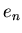in that equation with the difference between the convolution of the reference signal,with the coefficientsand the actual filter input data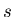: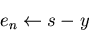(10)Next: Conclusion Up: number9 Previous: The RLS filter
Skip Carter 2008-08-20